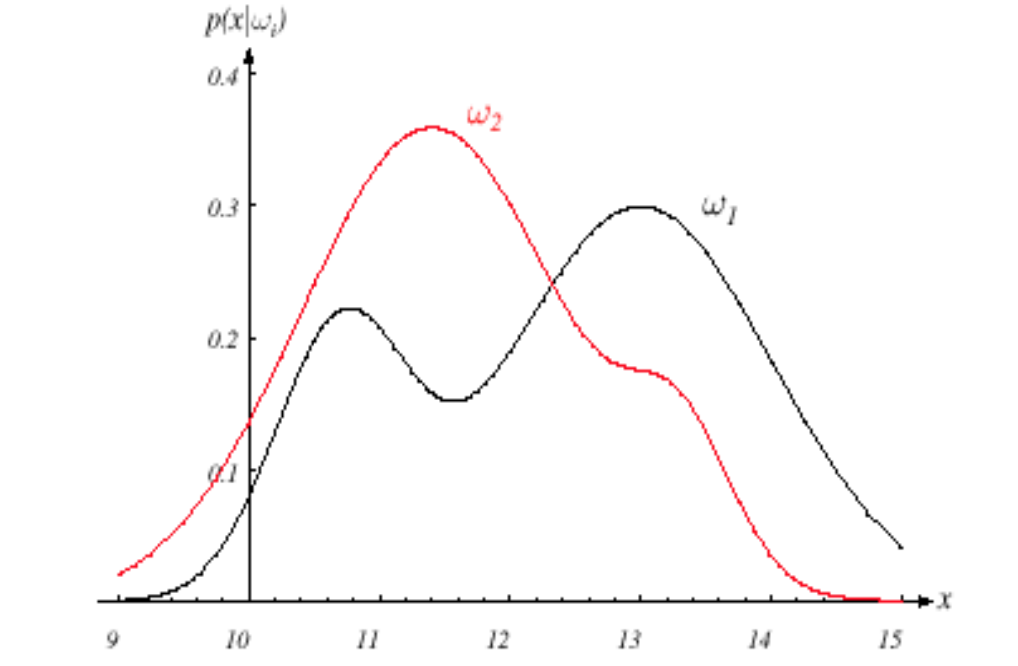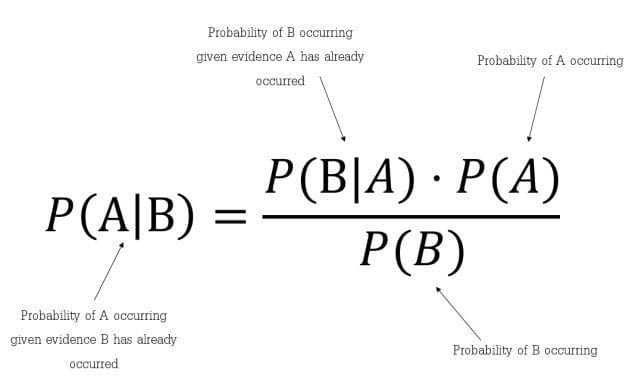This article was published as a part of the Data Science Blogathon

## Introduction

Bayesian decision theory refers to the statistical approach based on tradeoff quantification among various classification decisions based on the concept of Probability(Bayes Theorem) and the costs associated with the decision.

It is basically a classification technique that involves the use of the Bayes Theorem which is used to find the conditional probabilities.

In Statistical pattern Recognition, we will focus on the statistical properties of patterns that are generally expressed in probability densities (pdf’s and pmf’s), and this will command most of our attention in this article and try to develop the fundamentals of the Bayesian decision theory.

## Prerequisites

Random Variable

A random variable is a function that maps a possible set of outcomes to some values like while tossing a coin and getting head H as 1 and Tail T as 0 where 0 and 1 are random variables.

Bayes Theorem

The conditional probability of A given B, represented by P(A | B) is the chance of occurrence of A given that B has occurred.

P(A | B) = P(A,B)/P(B) or

By Using the Chain rule, this can also be written as:

P(A,B) = P(A|B)P(B)=P(B|A)P(A)

P(A | B) = P(B|A)P(A)/P(B)    ——-  (1)

Where, P(B) = P(B,A) + P(B,A’) = P(B|A)P(A) + P(B|A’)P(A’)

Here, equation (1) is known as the Bayes Theorem of probability

Our aim is to explore each of the components included in this theorem. Let’s explore step by step:

(a) Prior or State of Nature:

• Prior probabilities represent how likely is each Class is going to occur.
• Priors are known before the training process.
• The state of nature is a random variable P(wi).
• If there are only two classes, then the sum of the priors is P(w1) + P(w2)=1, if the classes are exhaustive.

(b) Class Conditional Probabilities:

• It represents the probability of how likely a feature x occurs given that it belongs to the particular class. It is denoted by,   P(X|A) where x is a particular feature
• It is the probability of how likely the feature x occurs given that it belongs to the class wi.
• Sometimes, it is also known as the Likelihood.
• It is the quantity that we have to evaluate while training the data. During the training process, we have input(features) X labeled to corresponding class w and we figure out the likelihood of occurrence of that set of features given the class label.

(c) Evidence:

• It is the probability of occurrence of a particular feature i.e. P(X).
• It can be calculated using the chain rule as,  P(X) = Σin P(X | wi) P(wi)
• As we need the likelihood of class conditional probability is also figure out evidence values during training.

(d) Posterior Probabilities:

• It is the probability of occurrence of Class A when certain Features are given
• It is what we aim at computing in the test phase in which we have testing input or features (the given entity) and have to find how likely trained model can predict features belonging to the particular class wi.

For a better understanding of the above theory, we consider an example

Problem Description

Suppose we have a classification problem statement where we have to classify among the object-1 and object-2 with the given set of features X = [x1, x2, …, xn]T.

Objective

The main objective of designing a such classifier is to suggest actions when presented with unseen features, i.e, object not yet seen i.e, not in training data.

In this example let w denotes the state of nature with w = w1 for object-1 and w = w2 for object-2. Here, we need to know that in reality, the state of nature is so unpredictable that we generally consider that was variable that is described probabilistically.

Priors

• Generally, we assume that there is some prior value P(w1) that the next object is object-1 and P(w2) that the next object is object-2. If we have no other object as in this problem then the sum of their prior is 1 i.e. the priors are exhaustive.
• The prior probabilities reflect the prior knowledge of how likely we will get object-1 and object-2. It is domain-dependent as the prior may change based on the time of year they are being caught.

It sounds somewhat strange and when judging multiple objects (as in a more realistic scenario) makes this decision rule stupid as we always make the same decision based on the largest prior even though we know that any other type of objective also might appear governed by the leftover prior probabilities (as priors are exhaustive in nature).

Consider the following different scenarios:

• If P(ω1)>>> P(ω2), our decision in favor of ω1 will be correct most of the time we predict.
• But if P(ω1)= P(ω2), half probable of our prediction of being right. In general, the probability of error is the minimum of P(ω1) and P(ω2), and later in this article, we will see that under these conditions no other decision rule can yield a larger probability of being correct.

Feature Extraction process (Extract feature from the images)

A suggested set of features- Length, width, shapes of an object, etc.

In our example, we use the width x, which is more discriminatory to improve the decision rule of our classifier. The different objects will yield different variable-width readings and we usually see this variability in probabilistic terms and also we consider x to be a continuous random variable whose distribution depends on the type of object wj, and is expressed as p(x|ωj) (probability distribution function pdf as a continuous variable) and known as the class-conditional probability density function. Therefore,

The pdf p(x|ω1) is the probability density function for feature x given that the state of nature is ω1 and the same interpretation for p(x|w2).Fig. Picture Showing pdf for both classes

Suppose that we are well aware of both the prior probabilities P(ωj) and the conditional densities p(x|ωj). Now, we can arrive at the Bayes formula for finding posterior probabilities:Fig. Formula of Bayes Theorem

Bayes’ formula gives us intuition that by observing the measurement of x we can convert the prior P(ωj) to the posteriors, denoted by P(ωj|x) which is the probability of ωj given that feature value x has been measured.

p(x|ωj) is known as the likelihood of ωj with respect to x.

The evidence factor, p(x), works as merely a scale factor that guarantees that the posterior probabilities sum up to one for all the classes.

Bayes’ Decision Rule

The decision rule given the posterior probabilities is as follows

If P(w1|x) > P(w2|x) we would decide that the object belongs to class w1, or else class w2.

Probability of Error

To justify our decision we look at the probability of error, whenever we observe x, we have,

P(error|x)= P(w1|x) if we decide w2, and P(w2|x) if we decide w1

As they are exhaustive and if we choose the correct nature of an object by probability P then the leftover probability (1-P) will show how probable is the decision that it the not the decided object.

We can minimize the probability of error by deciding the one which has a greater posterior and the rest as the probability of error will be minimum as possible. So we finally get,

P(error|x) = min [P(ω1|x),P(ω2|x)]

And our Bayes decision rule as,

Decide ω1 if P(ω1|x) >P(ω2|x); otherwise decide ω2

This type of decision rule highlights the role of the posterior probabilities. With the help Bayes theorem, we can express the rule in terms of conditional and prior probabilities.

The evidence is unimportant as far as the decision is concerned. As we discussed earlier it is working as just a scale factor that states how frequently we will measure the feature with value x; it assures P(ω1|x)+ P(ω2|x) = 1.

So by eliminating the unrequired scale factor in our decision rule we have, the similar decision rule by Bayes theorem as,

Decide ω1 if p(x|ω1)P(ω1) >p(x|ω2)P(ω2); otherwise decide ω2

Now, let’s consider 2 cases:

• Case-1: If class conditionals are equal i.e, p(x|ω1)= p(x|ω2), then we arrive at our premature decision rule governed by just priors.
• Case-2: On the other hand, if priors are equal i.e, P(ω1)= P(ω2) then the decision is entirely based on class conditionals p(x|ωj).

This completes our example formulation!

## Generalization of the preceding ideas for Multiple Features and Classes

Bayes classification: Posterior, likelihood, prior, and evidence

P(wi | X)= P(X | wi) P(wi) / P(X)

Posterior = Likelihood* Prior/Evidence

We now discuss those cases which have multiple features as well as multiple classes,

Let the Multiple Features be X1, X2, … Xn and Multiple Classes be w1, w2, … wn, then:

P(wi | X1, …. Xn) = P(X1,…. , Xn|wi)*P(wi)/P(X1,… Xn)

Where,

Posterior = P(wi | X1, …. Xn)

Likelihood = P(X1,…. , Xn|wi)

Prior = P(wi)

Evidence = P(X1,… ,Xn)

In cases of the same incoming patterns, we might need to use a drastically different cost function, which will lead to different actions altogether. Generally, different decision tasks may require features and yield boundaries quite different from those useful for our original categorization problem.

So, In the later articles, we will discuss the Cost function, Risk Analysis, and decisive action which will further help to understand the Bayes decision theory in a better way.

## End Notes

If you liked this and want to know more, go visit my other articles on Data Science and Machine Learning by clicking on the Link

Something not mentioned or want to share your thoughts? Feel free to comment below And I’ll get back to you.

## Chirag Goyal

Currently, I am pursuing my Bachelor of Technology (B.Tech) in Computer Science and Engineering from the Indian Institute of Technology Jodhpur(IITJ). I am very enthusiastic about Machine learning, Deep Learning, and Artificial Intelligence.

The media shown in this article are not owned by Analytics Vidhya and is used at the Author’s discretion.